The average child learns about percentages in fifth or sixth grade. Most are introduced to the concept as parts of a hundred, for example, 100% of an item represents the whole, half would be half of one hundred, or 50/100, representing 50%. While most people can grasp this simple concept, calculating percentages of other numbers might prove more difficult for some. But knowing how to work with percentages is vital to go shopping, buy groceries and figure out discounts. Here are a few essential facts that can help students and consumers calculate percentages.

## Percentage and Fractions Connection

Knowing the connection between fractions, decimals, and percentages will help students to understand how to calculate percentages when given a fraction or decimal. A percentage and fractions generally work best when equating them with 100 and taking that hundred to be the whole. A percentage can be converted to a percentage by first dividing the top number by the bottom number and then multiplying by one hundred. For example, the fraction 2/5, when divided, equals 0.4. Multiplied by one hundred, this equals 40. Therefore, 2/5 is equal to 40%.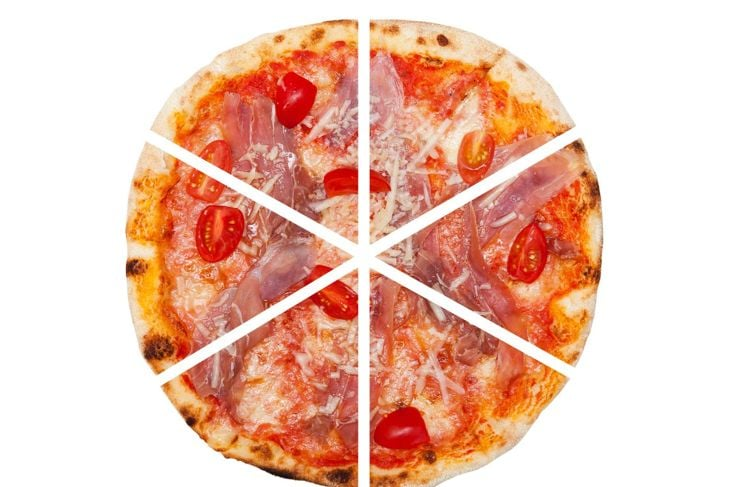Issaurinko / Getty Images

## Percentage of a Whole

To determine the percentage of one item within a group of items, the student first calculates how many items there are in total. For example, if students have a bowl of identical candies in different colors, they can count the number of candies to find out how many are in total. That number then represents 100%. Students can then count the number of candies that they wish to use to calculate the percentage and use that as the numerator (top number) in a fraction with the denominator (bottom number) representing the total. Once the fraction is constructed, they can divide it and multiply the answer by 100 to get the percentage.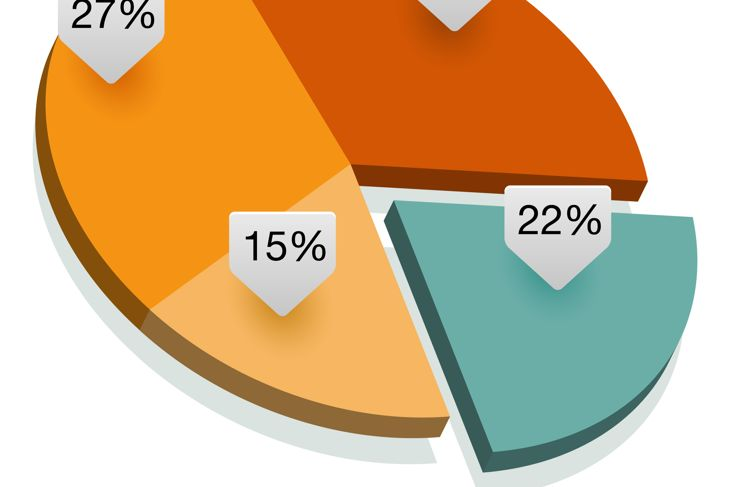exdez / Getty Images

## Example Problem for Percentage of a Whole

There is a bowl of hard candies sitting on a table. Fifteen are peppermint, ten are cinnamon, and 35 are Butterscotch. How would students go about finding out what percentage of the candies are peppermint? First, they need to add each type of candy together to find out the total number of candies in the bowl. This would be 15 (peppermint) + 10 (cinnamon) + 35 (butterscotch) = 60 total pieces. Therefore, 60 represents 100%. We want to know the percentage of peppermint candies. We know we have 15 of them. The number 15 becomes the numerator of a fraction, and 60 is the denominator of the fraction. Fifteen divided by 60 equals 0.25. Multiplied by 100, that makes 25, so 25% of the candies are peppermint.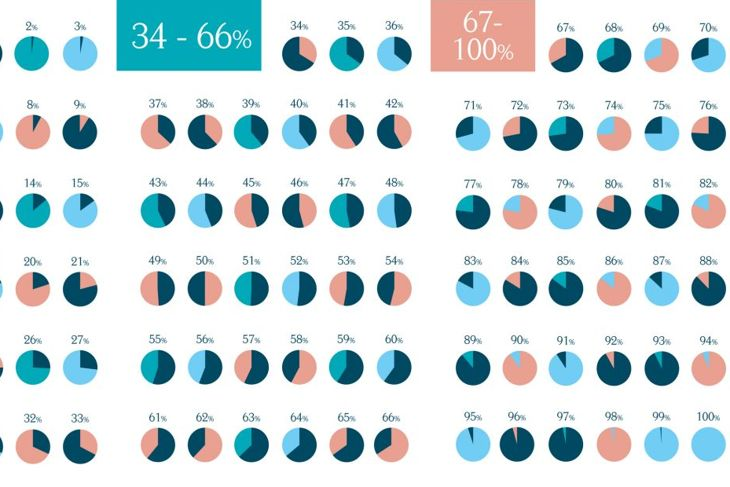## Working Backwards from Percentage

Sometimes it is necessary to calculate a number when given a percentage value. Consumers will run into this situation when figuring out how much to tip a server or how much they'll pay in simple interest. It can also be used to determine the amount of sales tax they'll have to pay for an item. A potential example might be figuring out how much of a tip to leave on a \$35 meal if the standard tip is 15%.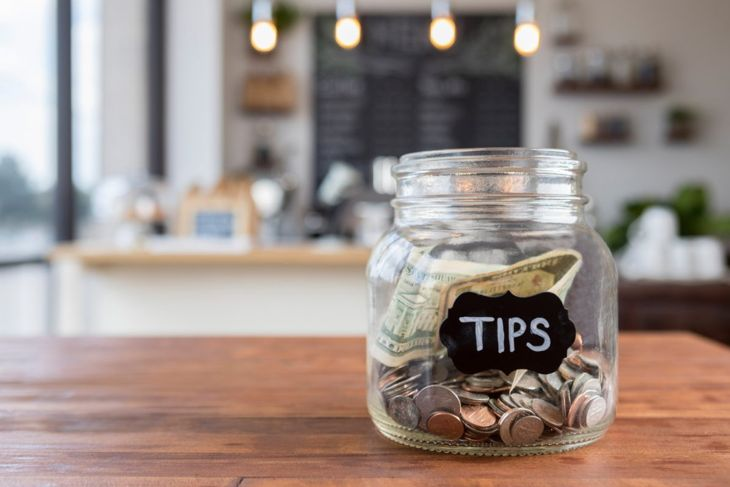## Backward Percentage Example

Using the previous example, assume that a meal at a fine restaurant costs \$35. The standard tip for a meal is 15%. How much money should the diners put aside as a tip? To figure this question out, students will need to calculate 15% of \$35. To do this, convert 15% into a decimal. Fifteen percent is equivalent to 15/100, so insert a decimal point two spaces to the left, leaving .15 as the result. Then, multiply this by the amount of the meal (35). The answer is 35 x .15 = 5.25. Therefore, the tip should be \$5.25.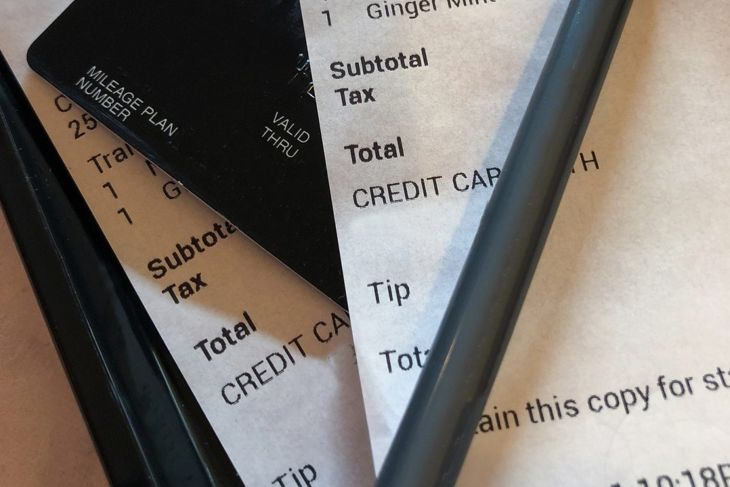## Calculating Discounts

When shopping for the best deal, it is often necessary to calculate the amount of a discount. Shopping centers are very good at marketing a sale as a great deal when in actuality the final cost isn't much better than the retail price. Knowing how to calculate a discount can save shoppers a lot of hassle and heartache. As an example, what is the final cost of a \$55 shirt if it is 35% off?## Discount Example Method One

Using the previous example, what is the final cost of a \$55 shirt if it is 35% off. To figure out the answer, consumers can take two approaches. The first method is to calculate the dollar amount of the discount and subtract it from the initial price. To do that, figure out what 35% of \$55 is (remember, this involves moving the decimal point two spaces to the left in the percentage (35%= .35) and then multiply by \$55. This equals .35 x 55 = \$19.25. So the discount is \$19.25. This amount should be subtracted from the initial cost to find the final price. So, \$55.00-\$19.25 = \$35.75. The final price of this shirt is \$35.75.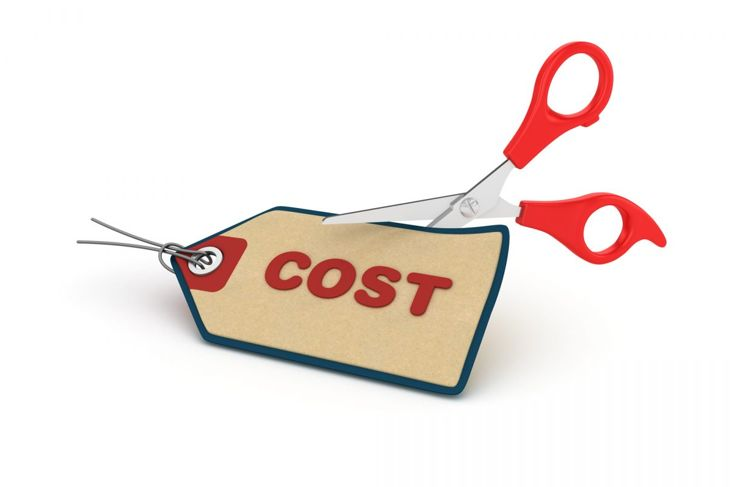porcorex / Getty Images

## Discount Example Method Two

What is the final cost of a \$55 shirt if it is 35% off? The second method for figuring this answer out is to figure out the percentage of the price that they will be paying after subtracting the discount and then multiply that by the price of the shirt. This is often easier and more direct. Since the shirt is 35% off, that means consumers will be paying 35% less than the full amount (100%). So they will be paying 100%-35% = 65% of the original cost. Following, 65% of \$55 is .65 x \$55 = \$35.75, which is the same answer consumers find using Method One.miakievy / Getty Images

## Finding the Amount of Interest Paid on a Loan

Homeowners often need to know how much interest they will be paying for a home or auto loan. For example, the average US auto loan APR interest rate in 2018 is 4.21% on a 60 month loan. Imagine purchasing a \$23,000 auto at this standard 4.21% annually. How much interest does the consumer have to pay at the end of the first year? To find the answer, simply convert the percentage to a percentage by moving the decimal point two spaces to the left (the equivalent of multiplying by .01). This leaves .0421. This amount is multiplied by the cost of the vehicle: 23,000 x .0421 = 968.3. So at the end of the first year, the interest paid is \$968.30.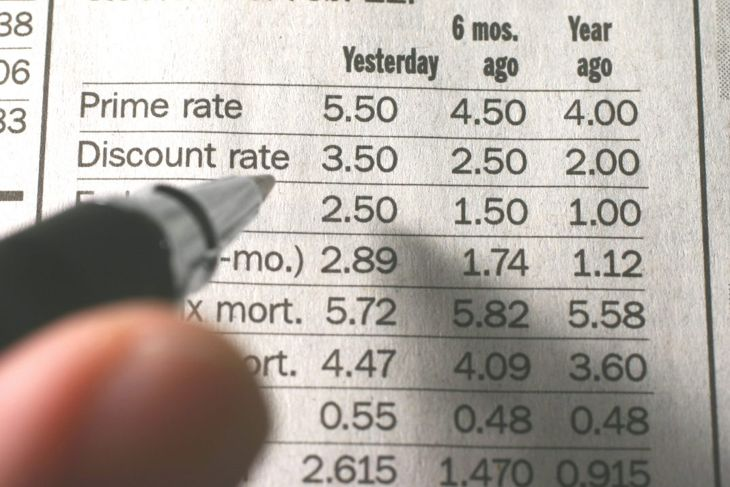RBFried / Getty Images

## The Importance of Percentages

Learning how to work with percentages is an important skill to develop for anyone who plans to enter the financial pool. Without a basic knowledge of percentages and how to manipulate them, it is difficult for consumers to make wise choices when shopping or spending money in general.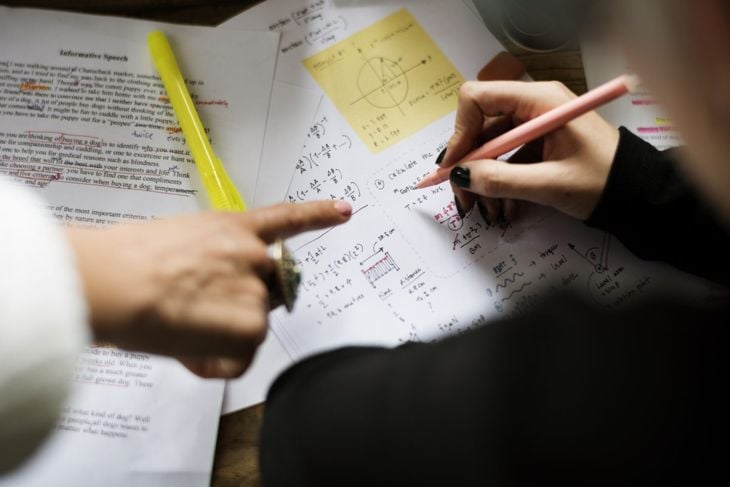Rawpixel / Getty Images